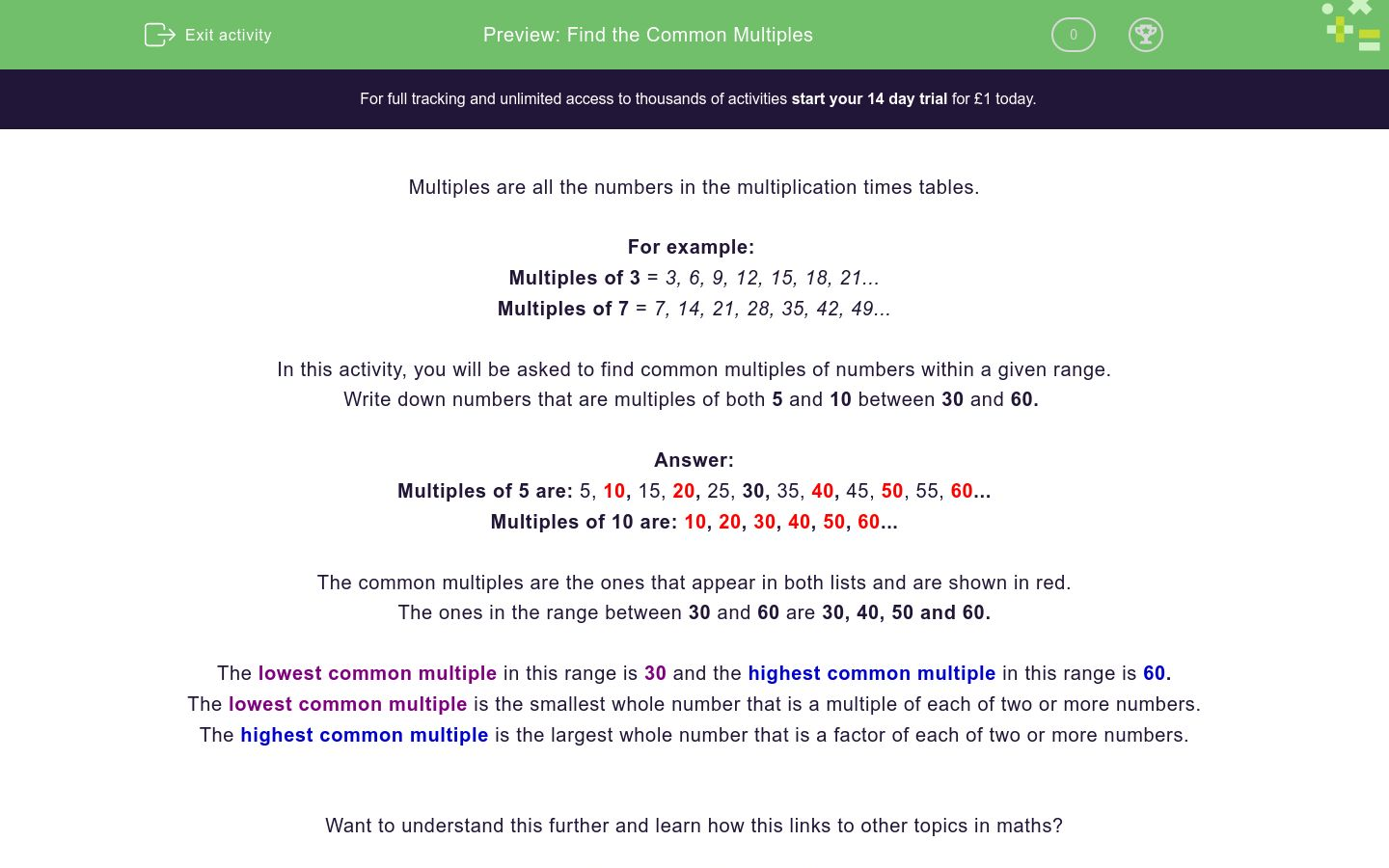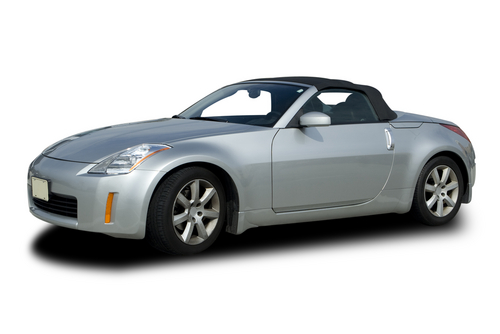# Find the Common Multiples

In this worksheet, students will find the common multiples of a variety of numbers, using knowledge of their multiplication tables.Key stage:  KS 2

Curriculum topic:   Number: Addition, Subtraction, Multiplication and Division

Curriculum subtopic:   Identify Common Factors, Multiples and Prime Numbers

Difficulty level:### QUESTION 1 of 10

Multiples are all the numbers in the multiplication times tables.

For example:

Multiples of 3 = 3, 6, 9, 12, 15, 18, 21...

Multiples of 7 = 7, 14, 21, 28, 35, 42, 49...

In this activity, you will be asked to find common multiples of numbers within a given range.

Write down numbers that are multiples of both 5 and 10 between 30 and 60.

Multiples of 5 are: 5, 10, 15, 20, 25, 30, 35, 40, 45, 50, 55, 60...

Multiples of 10 are: 10, 20, 30, 40, 50, 60...

The common multiples are the ones that appear in both lists and are shown in red.

The ones in the range between 30 and 60 are 30, 40, 50 and 60.

The lowest common multiple in this range is 30 and the highest common multiple in this range is 60.

The lowest common multiple is the smallest whole number that is a multiple of each of two or more numbers.

The highest common multiple is the largest whole number that is a factor of each of two or more numbers.

Want to understand this further and learn how this links to other topics in maths?

Why not watch this video?

What is the lowest common multiple of 12 and 18?

48

2

36

72

80

32

What is the lowest common multiple of 36 and 60?

48

2

36

72

80

32

Can you find the lowest common multiple of 5 and 7?

Remember to list the multiples for each number out first.

Can you match the multiples of 12 and 9 correctly?

This should then show you which is the lowest common multiple for the two numbers.

## Column B

Multiple of 12
36
Multiple of 9
36
Multiple of 12
24
Multiple of 9
48
Multiple of 12
27
Multiple of 9
45

What is the lowest common multiple of 11 and 8?

Remember to write a list of multiples for each number to help you.

Write down two numbers between 50 and 150 that are multiples of both 7 and 9.

Write down two numbers between 40 and 80 that are multiples of both and 8.

Write down two numbers between 25 and 75 that are multiples of both and 7.

Sally and Jasmine were each given a piece of ribbon of equal length.

Sally cuts hers into equal lengths of 2m, while Jasmine cuts her ribbon into equal lengths of 5m.

If there were no remainder in both cases, find the shortest possible length of ribbon given to them.  Give your answer without the units.On a track for remote-controlled racing cars, racing car A completes the track in 28 seconds, while racing car B completes it in 24 seconds.

If they both start at the same time, after how many seconds will they be side by side again? Give your answer in seconds.• Question 1

What is the lowest common multiple of 12 and 18?

36
EDDIE SAYS
The best way to work out the answer for this type of question is to list the multiples for each number: Multiples of 12 are: 12, 24, 36, 48, 60. Multiples of 18 are: 18, 36, 54, 72, 90. It is then easy for us to spot that the lowest common multiple is 36.
• Question 2

What is the lowest common multiple of 36 and 60?

EDDIE SAYS
So, from completing the blank spaces above, hopefully, you have seen that the lowest common multiple of 36 and 60 is 180. Multiples of 36 are: 36, 72, 108, 144, 180 Multiples of 60 are: 60, 120, 180, 240
• Question 3

Can you find the lowest common multiple of 5 and 7?

Remember to list the multiples for each number out first.

35
EDDIE SAYS
The lowest common multiple for 5 and 7 is 35. We can check this is right by listing the multiples for each number: The multiples of 5 are: 5, 10, 15, 20, 25, 30, 35 The multiples of 7 are: 7, 14, 21, 28, 35
• Question 4

Can you match the multiples of 12 and 9 correctly?

This should then show you which is the lowest common multiple for the two numbers.

## Column B

Multiple of 12
36
Multiple of 9
36
Multiple of 12
24
Multiple of 9
27
Multiple of 12
48
Multiple of 9
45
EDDIE SAYS
How did you get on? Here is a list of the multiples for 12 and 9: Multiples of 12 are: 12, 24, 36, 48, 60 Multiples of 9 are: 9, 18, 27, 36, 45 When we look closely at the two lists, we can find that the lowest common multiple is 36. Are you feeling more confident?
• Question 5

What is the lowest common multiple of 11 and 8?

Remember to write a list of multiples for each number to help you.

88
EDDIE SAYS
Did you find it? Here is a list of the multiples to help us spot the correct answer Multiples of 8 are: 8, 16, 24, 32, 40, 48, 56, 64, 72, 80, 88... Multiples of 11 are: 11, 22, 33, 44, 55, 66, 77, 88... From this, we can easily spot that the lowest common multiple of 8 and 11 is 88.
• Question 6

Write down two numbers between 50 and 150 that are multiples of both 7 and 9.

EDDIE SAYS
That was a tricky one! The lowest common multiple is 63 as 9 x 7 = 63. The highest common multiple in the given range is 126 as 9 x 14 = 126 and 7 x 18 is 126. The best way to calculate the answer is to keep counting using knowledge of your times' tables.
• Question 7

Write down two numbers between 40 and 80 that are multiples of both and 8.

EDDIE SAYS
That was more straightforward and within our usual times table range. The lowest common multiple is 48 as 6 x 8 = 48. The highest common multiple in the given range is 72 as 9 x 8 = 72 and 12 x 6 is 72.
• Question 8

Write down two numbers between 25 and 75 that are multiples of both and 7.

EDDIE SAYS
Again, using our times table knowledge will help us to solve questions like these. The lowest common multiple is 28 as 7 x 4 = 28 and the highest common multiple in the given range is 56 as 4 x 14 = 56 and 7 x 8 is 56. Is this becoming less daunting?
• Question 9

Sally and Jasmine were each given a piece of ribbon of equal length.

Sally cuts hers into equal lengths of 2m, while Jasmine cuts her ribbon into equal lengths of 5m.

If there were no remainder in both cases, find the shortest possible length of ribbon given to them.  Give your answer without the units.10
10 m
EDDIE SAYS
This was a tricky worded problem that needed you to find the lowest common multiple of 2 and 5. Using your times tables, you soon reach the lowest common multiple of 10, leaving the answer of 10m.
• Question 10

On a track for remote-controlled racing cars, racing car A completes the track in 28 seconds, while racing car B completes it in 24 seconds.

If they both start at the same time, after how many seconds will they be side by side again? Give your answer in seconds.168
168s
168 s
168 seconds
EDDIE SAYS
The two racing cars will be side by side again after 168 seconds or 2 minutes and 48 seconds. We had to find the lowest common multiple. Multiples of 24: 24, 48, 72, 96, 120, 144 and 168. Multiples of 28: 28, 56, 84 and 168. 24 x 7 = 168 28 x 4 = 168 Great focus! That's another activity ticked off. How about taking a break and attempting another one?
---- OR ----

Sign up for a £1 trial so you can track and measure your child's progress on this activity.

### What is EdPlace?

We're your National Curriculum aligned online education content provider helping each child succeed in English, maths and science from year 1 to GCSE. With an EdPlace account you’ll be able to track and measure progress, helping each child achieve their best. We build confidence and attainment by personalising each child’s learning at a level that suits them.

Get started